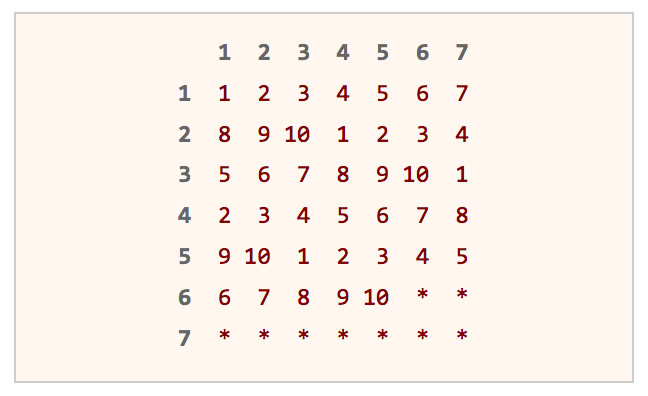# Given a function that generates random number from 1-7, write a function that generates random numbers from 1-10.

By | April 19, 2015

Question: You are given a function rand7() – that generates random numbers from 1-7. Write a function rand10() – that uses rand7() to generate random numbers from 1-10.

This appear to be one of those probabilistic analysis questions. You should be familiar with the concept of expected value, as it could be extremely helpful in probabilistic analysis.

Hint:
Assume you could generate a random integer in the range 1 to 49. How would you generate a random integer in the range of 1 to 10? What would you do if the generated number is in the desired range? What if it’s not?

Solution:
This solution is based upon Rejection Sampling. The main idea is when you generate a number in the desired range, output that number immediately. If the number is out of the desired range, reject it and re-sample again. As each number in the desired range has the same probability of being chosen, a uniform distribution is produced.

Obviously, we have to run rand7() function at least twice, as there are not enough numbers in the range of 1 to 10. By running rand7() twice, we can get integers from 1 to 49 uniformly. Why?##### A table is used to illustrate the concept of rejection sampling. Calling rand7() twice will get us row and column index that corresponds to a unique position in the table above. Imagine that you are choosing a number randomly from the table above. If you hit a number, you return that number immediately. If you hit a *, you repeat the process again until you hit a number.

Since 49 is not a multiple of tens, we have to use rejection sampling. Our desired range is integers from 1 to 40, which we can return the answer immediately. If not (the integer falls between 41 to 49), we reject it and repeat the whole process again.

```int rand10()
{
int row, col, idx;
do
{
row = rand7();
col = rand7();
idx = col + (row-1)*7;
}
while (idx > 40);

return 1 + (idx-1)%10;
}
```Author: nikoo28

a tech-savvy guy and a design buff...

## 7 thoughts on “Given a function that generates random number from 1-7, write a function that generates random numbers from 1-10.”

1.Simon

Is this correct? (rand7() – 1)/6*9 + 1
rand7() – 1 generates uniform distribution between 0 and 6
(rand7() – 1)/6 generates uniform distribution between 0 and 0.1
(rand7() – 1)/6*9 generates uniform distribution between 0 and 9
(rand7() – 1)/6*9 + 1 generates uniform distribution between 1 and 10

1.Simon

There is typo in my comment above, I correct my question as below:

Is this correct? (rand7() – 1)/6*9 + 1
rand7() – 1 generates uniform distribution between 0 and 6
(rand7() – 1)/6 generates uniform distribution between 0 and 1
(rand7() – 1)/6*9 generates uniform distribution between 0 and 9
(rand7() – 1)/6*9 + 1 generates uniform distribution between 1 and 10

2.Hiren

Couldn’t it be done as follows?
rand7() + (rand7() % 4)

1.Ryan

No, 0 will only be generated once when the numbers 1-7bbl under modulus 4

3.Igor

Hi, I wonder what’s the time complexity of this algorithm? Is it O(1)?

4.arulsubramaniam

Good one . I think , the question is written wrongly in the post . Please correct it.

`Question: There is an array A[N] of N numbers. You have to compose an array Output[N] such that Output[i] will be equal to multiplication of all the elements of A[N] except A[i]. Solve it without division operator and in O(n).`
1.nikoo28 Post author

Thanks for correcting the typo. :)
This happens, when you copy your previous question to keep the formatting changes. :P

This site uses Akismet to reduce spam. Learn how your comment data is processed.